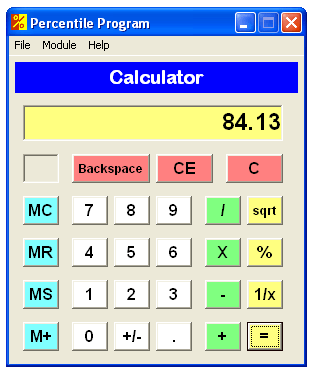# The Percentile Program

The Percentile Program handles all aspects of percentile calculations within the context of neuropsychological and psychological assessment. The Percentile Program will:

• Calculate percentiles when provided with raw scores, means, and standard deviations.
• Convert among percentiles, scaled scores, standard scores, t-scores, and z-scores.
• Perform inverse percentile calculations, where you begin with a percentile and work backward to calculate scaled scores, standard scores, t-scores, and z-scores.
• The Percentile Program also has an age calculator and a regular numerical calculator.

## Accuracy

The Percentile Program is highly accurate. The algorithm to calculate percentiles uses a polynomial approximation of the cumulative area under the normal curve. This algorithm is described in the "Handbook of Mathematical Functions with Formulas, Graphs & Mathematical Tables," edited by Milton Abramowitz and Irene A. Stegun (published by Dover Publications, 1972). The absolute error in this algorithm is less than 7.5 x 10-8, making these calculations accurate to about seven decimal places.

Inverse percentile calculations are accomplished with an approximation of the inverse normal cumulative distribution function. The absolute error in this algorithm is less than 1.15 x 10-9, making these calculations accurate to about eight decimal places.

## ScreenshotsRaw Scores ModuleScore Converter ModuleAge CalculatorNumerical Calculator

## Download

Updated January 14, 2018:

• Fixed a bug that caused the program to crash on 64-bit versions of Windows
• Converted the help file to PDF
• Other minor bug fixes and performance improvements

Click below to download the Percentile Program installation package (2.4 MB):